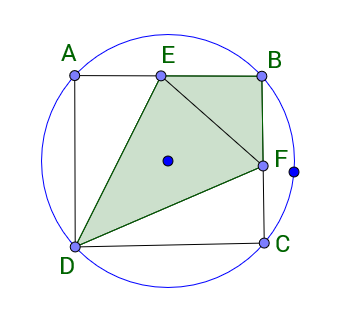Geometry Level 1$ABCD$ is a square inscribed in a circle of diameter $3\sqrt{2}$. $E$ and $F$ are the midpoints of $AB$ and $BC$, respectively. What is the area of the shaded region?

×